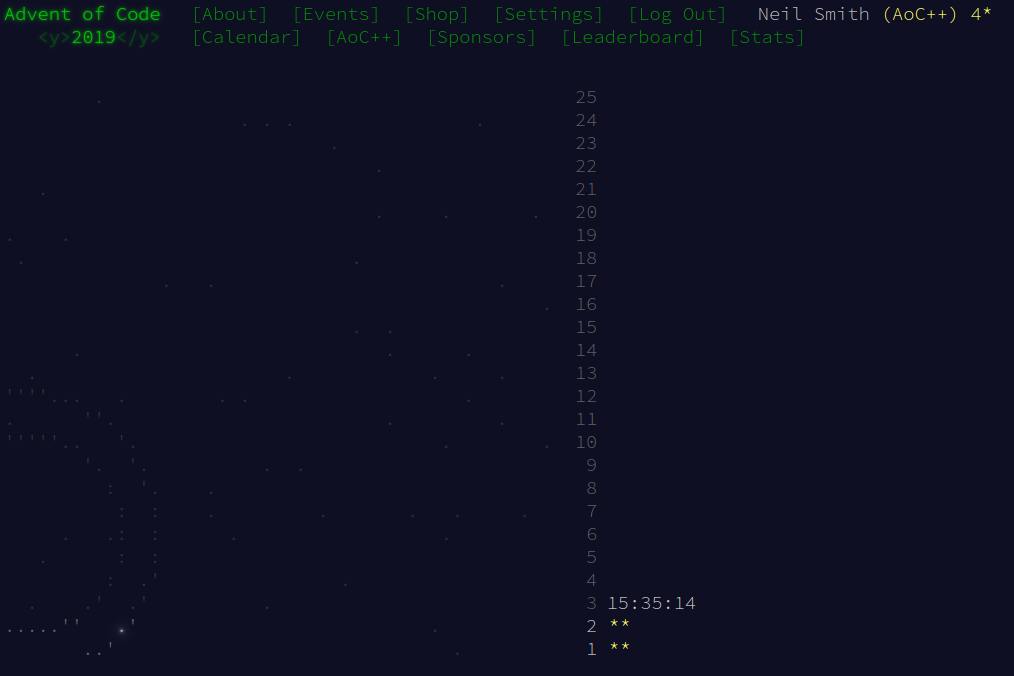December 2, 2019

# Advent of Code 2019 day 2I wasn't expecting to be writing mondaic State code on day 2! On the other hand, having done several Advent of Codes earlier, I had some previous code I could reuse. This problem is very similar to 2017 day 5 (code), but I'm keeping an eye on 2018 day 21 (code) for how this machine gets extended in future challenges.

## Parsing

Megaparsec continues to be overkill for the input, but still yields a very readable parser:

memoryP = integer sepBy comma


(The memory is a bunch of integers separated by commas.)

## Data structures

I'm not making a clever choice here, and representing the memory as a key-value store, where each key is a memory index and the value is the value at this index. This is called variously a dict, a Hash, an Object, and, in Haskell's case, a Map. (This is different from the map higher-order function.) As the indices are integers, I'm using the Data.IntMap.Strict library.

The machine as a whole is a record, comprising the memory and the instruction pointer:

type Memory = M.IntMap Int

data Machine = Machine { _memory :: Memory
, _ip :: Int
}
deriving (Show, Eq)


Given that we're doing lots of indirection for lookups and updates, I define a couple of utility functions as a bit of syntacic sugar.

-- prefix version of (!)
lkup k m = m!k

-- indirect lookup
(!>) m k = m!(m!k)

-- indirect insert
iInsert k v m = M.insert (m!k) v m


I use the standard State monad to run the machine. It saves plumbing the state of the machine through the computation. I could use something like a fold or even iterate to move from one machine state to another, but the monadic approach could well be neater in the long run.

A ProgrammedMachine is the monad, taking a machine, performing some operation on it, and returning both the modified machine and the return value. As there's no sensible return value, I use () for that.

runAll repeatedly runs a machine until the instruction has opcode 99. (I don't worry about trying to access locations outside the defined memory.) To run the machine, run one step, then continue running the whole thing.

runStep handles updating the machine, passing the detail of the operation to the perform function. There's one clause there for each operation we know about. Note the use of !> and iInsert to keep this code cleaner. perform is also responsible for updating the instruction pointer, following the hint in part 2 about future operations having different lengths.

type ProgrammedMachine = State Machine ()

runAll :: ProgrammedMachine
runAll = do m0 <- get
unless (lkup (_ip m0) (_memory m0) == 99)
do runStep
runAll

runStep :: ProgrammedMachine
runStep =
do m0 <- get
let mem = _memory m0
let ip = _ip m0
let (mem', ip') = perform (mem!ip) ip mem
put m0 {_ip = ip', _memory = mem'}

perform :: Int -> Int -> Memory -> (Memory, Int)
perform 1 ip mem = (iInsert (ip + 3) (a + b) mem, ip + 4)
where a = mem!>(ip + 1)
b = mem!>(ip + 2)
perform 2 ip mem = (iInsert (ip + 3) (a * b) mem, ip + 4)
where a = mem!>(ip + 1)
b = mem!>(ip + 2)


I run the machine by calling execState runAll machine: this runs the machine and returns its final state, discarding any value set by the monad.

## Part 1

That's enough to run the Part 1 challenge, with the added wrinkle of updating the memory before execution, and returning the value in memory location 0.

part1 machine = (_memory $execState runAll machine1202)!0 where machine1202 = machine { _memory = M.insert 1 12$ M.insert 2 2 $_memory machine }  ## Part 2 The part 2 challenge prompted some refactoring, extracting functions to set the noun and verb of the machine (machineNounVerb), to find the final location 0 result (machineOutput), and to glue those two parts together (nounVerbResult). nounVerbResult :: Int -> Int -> Machine -> Int nounVerbResult noun verb machine = machineOutput nvMachine where nvMachine0 = machineNounVerb machine noun verb nvMachine = execState runAll nvMachine0 machineNounVerb :: Machine -> Int -> Int -> Machine machineNounVerb machine noun verb = machine { _memory = M.insert 1 noun$ M.insert 2 verb $_memory machine } machineOutput :: Machine -> Int machineOutput machine = (_memory machine)!0  The actual solution to Part 2 is just an exhaustive search of all the noun-verb combinations, returning the first one that satisfies the requirement. (I trust the puzzle designer to have a unique solution.) part1 = nounVerbResult 12 2 part2Target = 19690720 part2 machine = noun * 100 + verb where (noun, verb) = head$ [(n, v) | n <- [0..99], v <- [0..99],
nounVerbResult n v machine == part2Target ]


That also allowed me to simplify the part1 code.

## Full code and refactoring

Around day 11, I decided to extract the "complete" Intcode interpreter into its own module. You can see the code I wrote on day 2 (and on Github),  and what it became once the interpreter was extracted (and on Github).